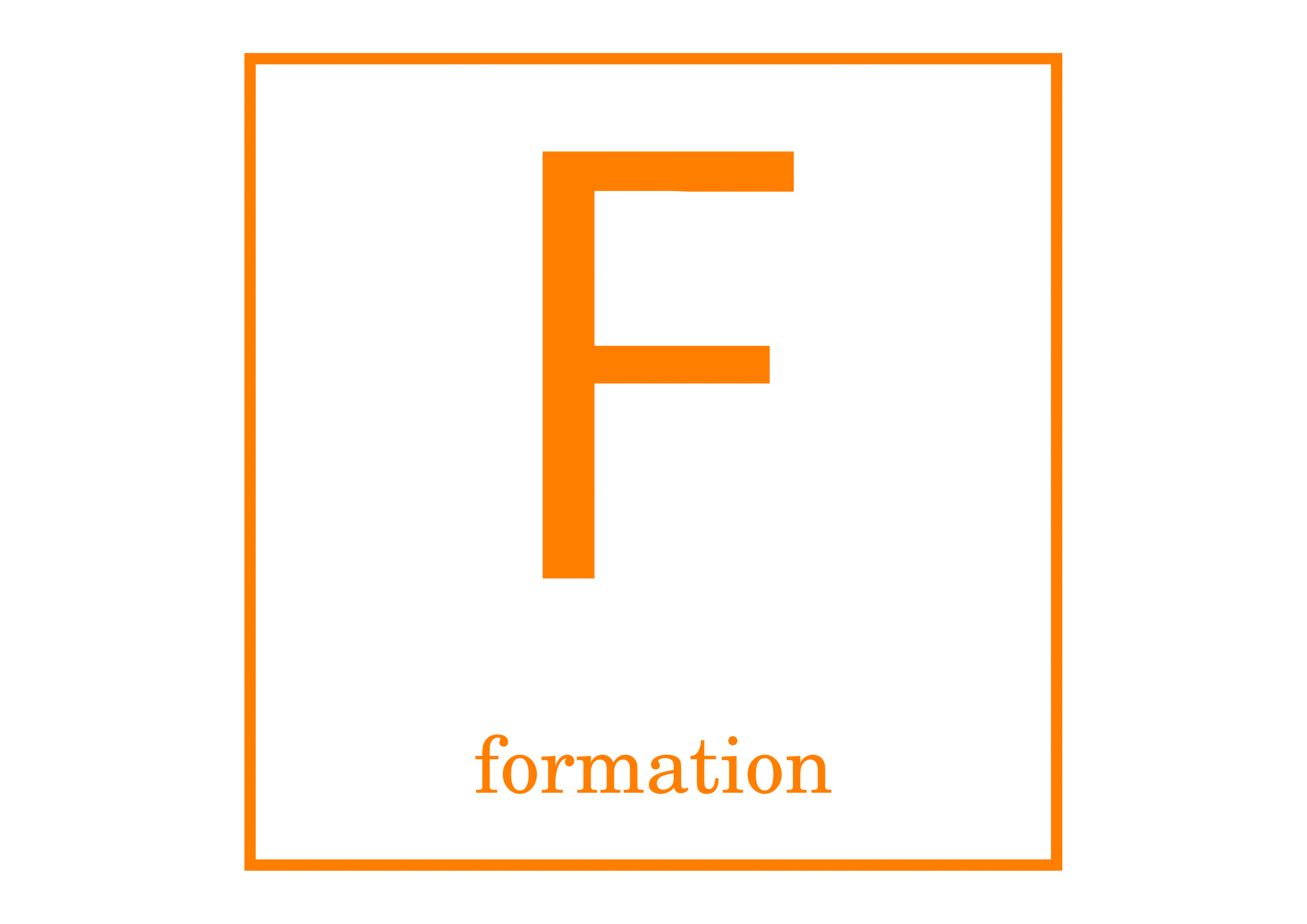# La physique à l'ENSCR|

# Surface tension

Objectives:

• To evaluate the surface tension of water, soapy water and mercury.
• To successfully project an image to improve the accuracy of the measurement.
• To verify Jurin's law, using a regression software.

## Surface tension

It costs energy to increase the surface of a liquid. A liquid thus adopts the form that minimizes its surface. If the only forces that apply to a liquid are those of surface tension, it can be shown that the area that minimizes the energy is a sphere. That is why soap bubbles are spherical.

Surface tension $\gamma$ measures the resistance to the increase in surface: if the surface S of a liquid varies by dS, then the energy of the liquid increases by $\mathrm{d}{E}=\gamma\,\mathrm{d}S$.

If now we consider a liquid film on a rectangular frame with one movable side, the liquid tries to minimize its surface by exerting a force on that side. The force required for the operator to maintain the area constant is $f=2\,\gamma\,\ell$ where $\ell$ is the length of the rod. The force is doubled through factor 2 because there are two interfaces: one above and one below.

### A droplet lying on its support

The shape of a droplet of liquid in equilibrium with its environment is due to the surface tension forces (aka capillary actions). The contact angle $\alpha$ is defined in the following figure. When it exceeds 90°, the liquid shows a low wettability.

The height of the droplet is $h=\sqrt{2\,\gamma\,\dfrac{1-\cos\alpha}{\mu\,g}}$ Therefore the surface tension can be calculated from the shape of the droplet: \begin{equation} \boxed{\gamma=\dfrac{\mu\,g\,h^2}{2(1-\cos\alpha)}} \label{eq:gammaforme} \end{equation}

### Direct measurement: Du Noüy ring method

For the direct measurement of the surface tension, we use a precision dynamometer as shown on the apparatus below, which gives the strength that the surface of the liquid exerts on the ring (until its detachment of this surface) along with the weight of the ring. This force approximately equals $f=4\pi\,r\,\gamma+m\,g$ at its maximum, since it is exerted on the inner and outer edges of the ring. \begin{equation} \boxed{\gamma=\dfrac{f-m\,g}{4\pi\,r}} \label{eq:gammadirect} \end{equation}

### Jurin's law

The rise and fall of a liquid within a thin capillary tube (aka capillary ascent) can be described by Jurin's law, provided that the tube is thin enough. The hydrostatic equilibrium results from the competition between gravity and surface tension. Jurin's law links the height of the ascent to the radius of the tube: $\boxed{h=\frac{2\,\gamma\,\cos\theta}{\mu\,g}\,\frac{1}{r}}$ $\gamma$ is the surface tension of the liquid and $\theta$ the contact angle.

### Preparation

Find the values of the surface tension of water and soapy water (on the internet).

Express uncertainty on surface tension $\gamma$, from formula \eqref{eq:gammaforme}, based on uncertainties on the droplet's height and angle. The density and the acceleration of gravity are precise enough in order to neglect their influence on the total uncertainty.

### Manipulations

#### A droplet lying on its support (45')

The following steps shall be repeated for a droplet of water and a soapy water droplet on a special material.

Note: for law \eqref{eq:gammaforme} to be valid, the droplet must be flat enough.

Make the image of the droplet on a screen using a lamp and a lens. Make sure you choose the right lens.

Reproduce the image of the droplet on a sheet of paper and project the image of a ruler to determine the enlargement of the lens.

Measure the height of the droplet and the contact angle (take the average value of the two or four visible angles). Assess the uncertainties on the height and angle.

Find the value of the surface tension of this droplet and its uncertainty. The density of mercury is $\mu=13\,600~\mathrm{kg.m^{-3}}$. The acceleration of gravity is $g=9{,}8~\mathrm{m.s^{-2}}$.

#### Du Noüy ring method (30')

Fill the small crystallizer with water.

Measure the weight of the ring with the dynamometer and eventually hide this weight using the sliding over-tube.

Slowly lower the laboratory scissor jack and write down the maximum strength as shown by the dynamometer, before the ring is pulled off the water.

Deduce the surface tension of water at room temperature and the uncertainty of the measure. Do not forget to deduct the weight of the ring.

Start again and add surfactant into the water (a few drops of dish soap).

Remove the ring after the experiment to preserve the spring of the dynamometer.

#### Jurin's law (45')

Choose the most appropriate lens and project the capillary tubes immersed in water onto a screen. It may be necessary to tap or slightly shake the tubes to help the water to rise.

Measure $h$ for all the tubes and perform a regression analysis to determine the relation between $h$ and $r$. Is the law of Jurin verified? Deduce the value of $\gamma\,\cos\theta$. Then, if possible, estimate the value of surface tension $\gamma$ and its uncertainty.

The radius of the tubes is, in mm: $r\in\{0{,}4\,;~0{,}6\,;~0{,}8\,;~1{,}6\}$.

Draw what the image of the tubes would be if you had put mercury instead of water.

## Pour en savoir plus...

1. Gouttes, bulles, perles et ondes. Belin, 2002.
2. Tension superficielle[en ligne]. Disponible sur femto-physique.fr
★★★

## Annexe : liste de matériel

• A special polymer support ;
• a quartz-iode light;
• two lens with differents focals and their supports
• a scissor jack ;
• a big crystallizer ;
• one milli-dynamometer ;
• one du Noüy ring ;
• four tubes for Jurin's law.